Controlling The Real World With Computers
::. Control And Embedded Systems .::

 Home Order Let me know what you think

This experiment shows how to use analog to control a PWM channel bi-directionally. The TIP120 darlington transistor (see TIP120.PDF) will be used here in a manner very similar to the way it was used in Experiment 10.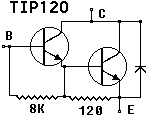The TO-220 package of the TIP120 is shown again below. Remember that the tab is connected to the collectors of the transistors, so if the heat sink is grounded the tab must be insulated from the heat sink but still conduct heat to it.A relay will be used to control the direction of the motor. The basic mechanical relay is an electromagnet that attracts one or more pole pieces when turned on. Each pole piece (usually shortened to the word "pole") is associated with one or more throw positions. Together they act as a switch.

The electromagnet is usually shown as a coil with the poles and throws above it. The poles are considered to be up and connected to the top throw positions when the magnet is off. The poles are considered to be pulled down when the magnet is turned on, connecting the poles to the lower throw positions. Thus, the upper throw positions are considered normally closed (NC) and the lower throw positions are considered normally opened (NO). A double pole double throw (DPDT) relay is shown schematically below: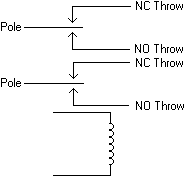The wiring of the motor, the TIP120, and the PPI is shown below as it was in Experiment 10, but with the addition of a relay for controlling direction. Two 1N4747A 20 volt zeners (see Experiment 4 for more on zeners) connected back-to-back protect the relay contacts from high voltage motor spikes of either polarity: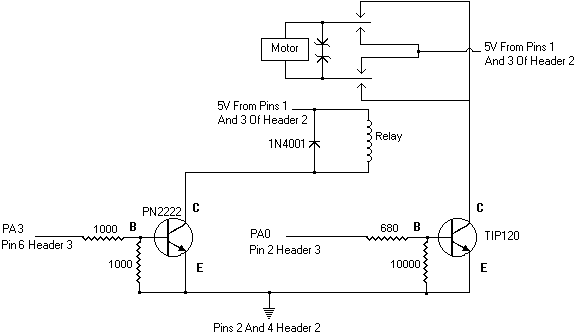The poles are up and connected to their normally closed positions when the relay's electromagnet is off. A close study of the diagram will show that this condition connects 5 volts to the bottom of the motor and the collectors of the TIP120 to the top of the motor. The opposite is true when the relay is turned on; the top of the motor connects to 5 volts and the bottom to the TIP120. The reversal changes the direction of the motor.

The relay's pins connect as follows. Notice the greater distance between the coil pins and the others: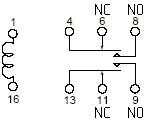The relay fits in the same space as a 16-pin IC. The above view is from the bottom, but the symmetry lines everything up from the top in basically the same manner. The numbering of the pins is kept the same as an IC even though some of the positions are not used. The poles are pins 4 and 13. They connect to the motor and the zeners. Connect 6 and 9 together, and 8 and 11 together. Connect 6 to either the 5 volt supply or to the collectors of the TIP120. Connect 8 to the place you didn't connect 6 to.

The relay will be driven with a PN2222 transistor: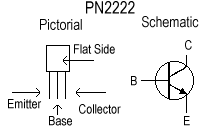The coil resistance of the relay I used is 125 ohms. It operates on 5 volts. Thus, from How To Read A Schematic, the current is 5/125 = .04 AMPS or 40ma. A transistor will be used to amplify the current from the 82C55 in order to control the relay.

The PN2222 transistor has a current gain (hfe) of at least 35 (see Experiment 4 for a definition of hfe). The hfe chart can be found on page 2 of PN2222.PDF. The 82C55 will provide 3 volts and 2.5ma of current (see the Electrical Specifications chart on page 17 of 82C55.PDF).

Recall from Experiment 4 that there is about .7 volts from the base to ground. If we use a 1K ohm resistor from the 82C55 to the base of the PN2222, the current will be
I = (3 - .7)/1000 = 2.3ma (see How To Read A Schematic).

The 1K to ground keeps down noise. Its current is .7/1000 = 700 microamps (millionths of an amp). This must be subtracted from the input current providing a net output current of
(((3 - .7)/1000) - (.7/1000)) * 35 = 56ma
(values in schematic)

The 82C55 will easily deliver the required current which will be amplified by a factor of 35, giving us 56ma, which is more than enough to drive the relay.

From TIP120.PDF it is found that the TIP120 has an hfe of 1000. If it is desired to drive a 1 AMP load, an input of 1/1000 = 1ma is needed. Recall from the schematic above that the emitter output of the first transistor is connected to the base of the second. The gain is the product of the gains of the two transistors. The base to emitter drop is now doubled to 1.4 V. That means we need an input resistor of
(3 - 1.4)/1ma = 1600 ohms

Another way to design the circuit is to provide the maximum available drive. The 82C55 can supply 2.5ma, so
(3 - 1.4)/2.5ma = 640 ohms

Since only 1ma is needed, let's go for about half of maximum and use 1200 ohms which is a standard value. This gives us
(3 - 1.4)/1200 = 1.333333ma

It's a good idea to have a resistor from base to ground to cut down on noise. We have .333333ma left to still give us 1ma drive current. Since there is 1.4 volts from base to emitter, the value of the resistor would be
1.4/.333333ma

A close standard value is 4.7K. We now have a net output current from the TIP120 of
(((3 - 1.4)/1200) - (1.4/4700)) * 1000 = 1.035460993 AMPS

If you want to go for maximum drive, try the standard 680 ohms value (always go high on this one). Now the current required of the 82C55 is
(3 - 1.4)/680 = 2.352941ma

That leaves 2.5 - 2.352941 = .147059ma for the noise suppression resistor. So,
1.4/.147059ma = 9519.988576

The closest standard value is 10K. The net drive current is now
((3 - 1.4/680) - (1.4/10000)) * 1000 = 2.212941176 AMPS
(values in schematic)

NOTE: Please be sure to read the Warranty And Disclaimer before working with the hardware!

The output control header module has been changed to modify the structure for setting up an analog channel:

 // outct11a.h ......... struct anachan { int status; int last_value; int current_value; int controls_PWM; int PWM_channel; int PWM_Direction_Channel; int arraynumber; int NoiseBand; }; ......... // end outct11a.h

The only element that has been added that is different from Experiment 10 is PWM_Direction_Channel. It has been added to provide a port number for controlling direction.

The following shows the changes to TurnOnAnalog(..) in the timer header and C modules:

 // timer11a.h ......... int TurnOnAnalog(int channel, // analog channel number int type, // 0=no digital control, 1=forward, 2=bi-directional int arraynumber, // output control array number int PWMPortNumber, // port bit for forward control int PWMDirectionPort, // port bit for direction control int NoiseBand); // current and last reading must differ by // this much for PWM change to take place ......... // end timer11a.h

 // timer11a.c ......... int TurnOnAnalog(int channel, // analog channel number int type, // 0=no digital control, 1=forward, 2=bi-directional int arraynumber, // output control array number int PWMPortNumber, // port bit for forward control int PWMDirectionPort, // port bit for direction control int NoiseBand) // current and last reading must differ by // this much for PWM change to take place { if(channel < 0 || channel > 7) return -1; AnnalogChannel[channel].status = START_CONVERSION; if(type) { AnnalogChannel[channel].controls_PWM = type; AnnalogChannel[channel].PWM_channel = PWMPortNumber; AnnalogChannel[channel].PWM_Direction_Channel = PWMDirectionPort; AnnalogChannel[channel].arraynumber = arraynumber; AnnalogChannel[channel].NoiseBand = NoiseBand; AnnalogChannel[channel].last_value = 0; } DA_Enabled = 1; return channel; } ......... // end timer11a.c

Some changes have been made to pwm(..) and PwmAndDirectionDuty(..) in the timer C module. The pwm(..) routine now simply turns the output off if any of the times are out of range. It also now handles the type 2 mode; the one used in this experiment. PwmAndDirectionDuty(..) is sped up a little when the forward and reverse duty cycles are the same as they would be in the case where a direction line is used:

The ConfigureOutput(..) output routine in the digital module has also been changed to handle type 2:

Several print lines are included in all of the modules for debugging purposes. Rem them out if you don't want to see all of the status printing.

To test the procedures, run the following and vary the offset control:

 // exper11a.c // exper11a.c #include "exper11a.h" int CheckAnalog(int analog_channel); enum { MainMotor, LED1, LED2, LASTSLOT }; void main(void) { int x; double dc,oldfreq,newfreq; oldfreq = 1193180.0/65536.0; printf("oldfreq = %f calling set_up_new_timer for 1K Hz\n",oldfreq); set_up_new_timer(1000.0); newfreq = get_frequency(); printf("new frequency = %fHz\n",newfreq); x = (int)InitializeAnalog(); printf("init ana = %X\n",x); // make everthing an output set_up_ppi(Aout_CUout_Bout_CLout); x = TurnOnAnalog(0, // analog channel number 2, // 0 = no digital control, 1 = uni-directional, 2 = bi-directional MainMotor, // output control array number PA0, // port bit for forward control PA3, // port bit for direction control 6); // current and last reading must differ by // this much for PWM change to take place Blink(LED1,PA1, .03, .02); Blink(LED2,PA2, .3, .2); printf("Blinking LED1=PA1=%d .03,.02; LED2=PA2=%d .3,.2\n",PA1,PA2); printf("TurnOnAnalog(PWM=PA0=%d, Direction=PA3=%d) = %d\n",PA0,PA3,x); printf("Press any key to continue then any key to quit -- no Ctr-C!\n"); getch(); while(!kbhit()) { if(!CheckAnalog(0)) break; } portaoff(); // be sure to restore the timer! restore_old_timer(); portaoff(); } int CheckAnalog(int anachan) { int SpeedValue,SetDirection; if(abs(AnalogChannel[anachan].current_value - AnalogChannel[anachan].last_value) > AnalogChannel[anachan].NoiseBand) { AnalogChannel[anachan].last_value = AnalogChannel[anachan].current_value; switch(AnalogChannel[anachan].controls_PWM) { case 1: // unidirectional UniPwmDuty(AnalogChannel[anachan].arraynumber, AnalogChannel[anachan].PWM_channel, (double)AnalogChannel[anachan].current_value/255.0); break; case 2: // bidirectional SpeedValue = AnalogChannel[anachan].current_value; // the low end of the values, from 0 to 127, are used for reverse // the high end values, from 128 to 255, are used for forward // for forward, the range is changed from 128 to 255 to 0 to 127 // for reverse, the range is flipped from 0 to 127 to 127 to 0 so // that the low end of the trimmer produces maximum reverse speed // the process ends up with 0 to 127 for both forward and reverse, // with direction set by the SetDirection variable if(SpeedValue > 127) // forward { SpeedValue-=128; // modify to 0 to 127 SetDirection = 1; // forward } else // reverse { SpeedValue = 127 - SpeedValue; // modify to 127 to 0 SetDirection = 2; // reverse } printf("AnalogChannel[%d] changed, now = %d, direction = %d\n" ,anachan,AnalogChannel[anachan].last_value,SetDirection); printf("SpeedValue = %d /127 = %f\n" ,SpeedValue,(double)SpeedValue/127.0); printf("AnalogChannel[anachan=%d].PWM_channel = %d\n" ,anachan,AnalogChannel[anachan].PWM_channel); printf("AnalogChannel[%d].PWM_Direction_Channel = %d\n" ,anachan,AnalogChannel[anachan].PWM_Direction_Channel); return PwmAndDirectionDuty(AnalogChannel[anachan].arraynumber, SetDirection, AnalogChannel[anachan].PWM_channel, AnalogChannel[anachan].PWM_Direction_Channel, (double)SpeedValue/127.0, (double)SpeedValue/127.0); break; } // end switch(AnalogChannel[anachan].controls_PWM) return 1; } // end if(abs(AnalogChannel[anachan].current_value...) } // end exper11a.c

If all conditions are met and the controls_PWM element is also set to 2, a call is made to PwmAndDirectionDuty(..) to change the duty cycle of the control channel located at arraynumber using the port designated by PWM_channel, as well as set the direction indicted by SetDirction using PWM_Direction_Channel.

Since the maximum value used for either forward or reverse is 127, the duty cycle is determined by dividing the processed input by 127.

Inputs from the Analog to Digital Converter and their modified values are illustrated below:

Input from Analog to Digital Converter: 0 127 128 255
After modification: 127   0   0 127
|   |   |   |_ maximum forward speed
|   |   |_____ minimum forward speed
|   |_________ minimum reverse speed
|_____________ maximum reverse speed